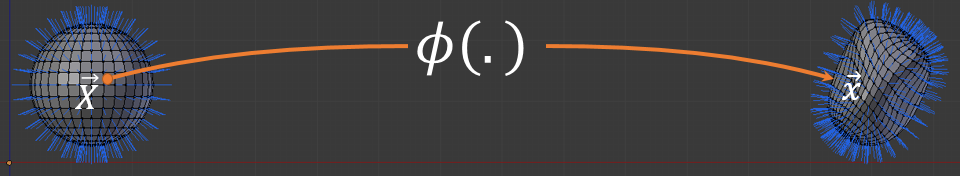# Transform normals given a deformation mapChanging the shape of a 3D mesh is a very common operation in computer graphics. For instance, rotations, translations etc. are very basic forms of mesh deformations. Of course more complicated algorithms exists to deform your mesh such as wrap deformers, linear blending skinning and so on... During the design of these algorithms you might face a question: how do I transform the normal of the original shape in rest pose to the deformed shape? This entry will give you some pointers to compute it.

Any deformations can be expressed as function $$\vec \phi (x, y, z) : \mathbb R^3 \rightarrow \mathbb R^3$$ the function takes in input a vertex position $$\vec {X}$$ in rest pose and outputs its deformed position $$\vec {x}$$. We call this function a deformation map, for instance:

Vector3 deform_vertex(Vector3 v){ return v + Vector3(5, 0, 0); }


Is a simple translation. It maps the rest shape to its translated version 5 units farther on the x axis. To compute the transformation of the normal associated to the rest mesh we "simply" (notice the quotes of sarcasm) need to compute the inverse transpose of the Jacobian of the deformation map $$\vec \phi$$:

$\left ({J \left ( \vec \phi (x, y, z) \right )}^{-1} \right )^{T}$

# The Jacobian

If you know what is a Jacobian skip this section.

What is the Jacobian of $$\vec { \phi }$$? In our case it is a $$3 \times 3$$ matrix. Therefore $${J(\vec \phi)}^{-1}$$ is the inverse of the $$3 \times 3$$ matrix $$J(\vec \phi)$$ and $${{J(\vec \phi)}^{-1}}^T$$ the transpose of the inverse. Note that $${{J(\vec \phi)}^{-1}}^T = {{J(\vec \phi)}^{T}}^{-1}$$ and so we can indifferently say the "inverse transpose" or the "transpose inverse".

Before I give the definition of the Jacobian I want to make sure we understand what is the function $$\vec \phi$$. The deformation map takes a point $$\vec X \in \mathbb R^3$$ and returns a new point $$\vec x \in \mathbb R^3$$. $$\vec \phi$$ is said to be a multivariate function since it takes several variables $$x$$, $$y$$ and $$z$$. More importantly it is a vector function since it returns a vector of three values:

$\vec \phi( \vec X ) = \begin{bmatrix} \phi_x(\vec X) \\ \phi_y(\vec X) \\ \phi_z(\vec X) \\ \end{bmatrix}$

We got all we need to define the Jacobian, here it is: it contains every first partial derivatives of $$\vec \phi$$ which are the column vectors $$\dfrac{\partial \vec \phi}{\partial x}$$, $$\dfrac{\partial \vec \phi}{\partial y}$$ and $$\dfrac{\partial \vec \phi}{\partial z}$$. Together they make the $$3 \times 3$$ Jacobian matrix:

$J (\vec \phi) = \begin{bmatrix} \dfrac{\partial \vec \phi}{\partial x} & \dfrac{\partial \vec \phi}{\partial y} & \dfrac{\partial \vec \phi}{\partial z} \end{bmatrix} = \begin{bmatrix} \dfrac{\partial \phi_x}{\partial x} & \dfrac{\partial \phi_x}{\partial y} & \dfrac{\partial \phi_x}{\partial z} \\ \dfrac{\partial \phi_y}{\partial x} & \dfrac{\partial \phi_y}{\partial y} & \dfrac{\partial \phi_y}{\partial z}\\ \dfrac{\partial \phi_z}{\partial x} & \dfrac{\partial \phi_z}{\partial y} & \dfrac{\partial \phi_z}{\partial z} \end{bmatrix} = \begin{bmatrix} \vec \nabla( \phi_x )^T \\ \vec \nabla( \phi_y )^T \\ \vec \nabla( \phi_z )^T \end{bmatrix}$

The  last expression is just another way to write the Jacobian using the transpose of the gradient vector.

# Understand: $${J(\vec \phi)}$$

Now that we defined the Jacobian lets understand why $$\vec n = {J \left ( \vec \phi (x, y, z) \right )}^{-T} . \vec N$$ or said another way: why the deformed normal $$\vec n$$ matches the rest normal $$\vec N$$ transformed by the inverse transposed Jacobian matrix?

This tutorial is unfinished I left here my outline for future improvements.

TODO

• Apply the Jacobian over a rigid transformation. Show what happens to the normal when applying jacobian
• Explain the inverse transpose trick trick using SVD which shows only scale gets inverted
• Study the meaning of the Jacobian matrix: it measures shape variations along x y and z
• // finish with a more complex example?

# Compute $$J$$ with finite differences

TODO:

One comment

Could you shed a bit more light on the : “inverse transpose trick”, a little tip to where to look in terms of “study the meaning of the jacobian matrix: direction of the surface variation in x y and z”, and the collider links just leads to 404 now.

Dmitry - 24-04-’19 22:42
(optional field, I won't disclose or spam but it's necessary to notify you if I respond to your comment)
All html tags except <b> and <i> will be removed from your comment. You can make links by just typing the url or mail-address.
Anti-spam question: